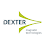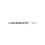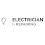## SAT Physics Magnetism - Force On Current-Carring Wires

FORCE ON CURRENT-CARRYING WIRES
Any combination of magnets will result in the field of one magnet (agent) creating a force on the second magnet (object). In the preceding section, fixed magnets and currents created forces on moving charges. What about other interactions? The field of a fixed magnet can create a force on a current-carrying wire, causing the wire to move. The field of one current-carrying wire can create a force on another current-carrying wire.

Fixed Magnets and Current-Carrying Wires
If a current-carrying wire passes through an external magnetic field, the field will create a force of magnetism on the wire, causing the wire to move. An external magnetic field is not the field of the object mentioned in the problem. The external magnetic field (X’s) shown in Figure 13.9 is caused by a set of fixed magnets that are positioned in front of and behind the page and cannot be shown in the diagram. This field acts as the agent that puts a force on the wire, the object. The field circling the wire is not shown since the wire does not act on itself.Figure 13.9. Current-carrying wire in an external magnetic field

The force acting on the wire is the product of the current in the wire (I), the length of the wire inside the fieldand the external magnetic fieldLike the force acting on moving charges, the formula contains sin θ.As long as the wire is perpendicular to the field (the most likely scenario on exams), the for­mula simplifies to the following when solving for vector magnitude.
Fb = ILB
The direction of this force is determined with the right-hand rule. Currents by definition are considered positive, so the right hand is needed. Use the left hand only if a problem specifically addresses a negative current or the actual electron flow. Since this problem involves force, use the straight finger version of the right-hand rule. The thumb is the direction of the moving charges, which in this case is the direction of the current, I.
The force of magnetism acting on a current-carrying wire is the basis of all electrical motors. In a motor, the wire is wrapped so that it forms many coils, which extends the length, L, and increases the magnetic force, FB, of the wire. The coils of wire are arranged so the mag­netic force can rotate the coils of wire continuously. The magnetic force in an electric motor converts electrical energy into mechanical energy.

Two Current-Carrying Wires
The magnetic field surrounding one current-carrying wire will create a force on a second nearby current-carrying wire. Consider two wires side by side, as shown in Figure 13.10. Wire 1 (agent) is surrounded by a magnetic field that creates a force of magnetism on wire 2 (object). This force will either attract wire 2 to wire 1 or repel it. You can also analyze the problem in reverse. Wire 2 is surrounded by a magnetic field. This field creates a force on wire
1. Newton’s third law dictates that the forces acting on both wires are equal and opposite.Figure 13.10. Focus on current-carrying wires

interacting wires involve two equations.one for the field of the first wire and one for the force acting on the second wire.The magnetic field (B1), of the first wire depends on the current in the first wire (I1), and the distance between the two wires (r). The force created on the second wire is the product of its current (I2), the length of wire 2 (L2), and the field of the first wire (B1).
Both versions of the right-hand rule are needed to solve for direction. The curled finger version is used to find the field direction of wire 1. The straight finger version is used to find the force on wire 2. To answer questions of this type quickly, memorize the fact that cur­rents in the same direction attract, and currents in the opposite direction repel. Be careful with this, as it is the opposite of other rules for attraction and repulsion. Normally, opposite attracts and like repels. However, with wires, currents in opposite directions repel and cur­rents in the same direction attract.

ELECTROMAGNETIC INDUCTION
The process of generating electricity is known as electromagnetic induction. This is the oppo­site of the electric motor. In a motor, a current carrying wire passing through a magnetic field receives a force causing it to move. In electromagnetic induction, a force is applied causing a loop of wire or a magnet to move in relation to each other. This induces a current to flow in the wire loop. Induction occurs when the amount of magnetic field, known as magnetic flux,Φ, experiences a change. The change in magnetic flux, ΔΦ, creates an electric potential that induces charges to flow though the loop. When an electric potential is induced, it is known as an emf, ε, rather than a voltage, and emf has the units of volts. Induction converts mechani­cal energy into electrical energy.

Magnetic Flux
Magnetic flux, Φ, can be thought of as the amount of magnetic field, B, passing straight through an area of space A as shown in Figure 13.11.Figure 13.11. Magnetic flux

Magnetic flux is the product of the magnetic field and the area through which it passes.
Φ = BA
In electromagnetic induction, the area used is where the magnetic field and the coil of wire overlap as shown by the gray areas in Figure 13.12.Figure 13.12. Electromagnetic induction

The coil of wire can have any shape, but is often rectangular or circular. It can consist of a single loop of wire or of many loops of wire. When many loops are shown, the wire coil may appear as a spiral helix where the area, A, is the area of one of the coils.
Inducing a current requires a change in flux, ΔΦ, and this fact is often the basis of conceptual questions. The change in flux can be determined as follows:From the equations, it is apparent that a change in flux results when either the magnetic field or the area change. This change can be accomplished in several ways.
• Moving the magnet and/or the coil toward each other increases the magnetic field, AB. See Figure 13.13.Figure 13.13. Coil and magnet moving toward each other
• Moving the magnet and/or the coil away from each other decreases the magnetic field,. See Figure 13.14.Figure 13.14. Coil and magnet moving away from each other
• Spinning a loop of wire in a magnetic field changes the area, ΔA See Figure 13.15.Figure 13.15. Rotating loop of wire in a magnetic field
• Moving the coil into or out of a magnetic field can change the area, ΔA. See Figure 13.16.Figure 13.16. Loop of wire moving into a magnetic field
Emf
Michael Faraday found that if the flux in a loop or coil of wire changes, then a current is cre­ated and flows through the loop or coil of wire. This process is known as electromagnetic induction. Changing the flux, ΔΦ, in a closed loop induces (creates) a potential (voltage) in the loop. This special induced voltage is known as an emf and is represented by the variable symbol, ε. It has the units of volts (V). Although it is known by another name, emf acts as any voltage does. The induced emf is the pressure that induces charges to move as a current in the loop of wire. Remember that a change in magnetic flux (a change in magnetic field or area) through a closed loop of conducting material is the necessary event that will induce an emf, ε, which in turn will induce a current to flow in the conducting loop.
The mathematical relationship between changing flux and the induced emf is described by Faraday’s law.The equation of Faraday’s law does include a minus sign. However, the minus sign is not needed to determine the magnitude of the induce emf and has been omitted from these equations. The importance and usage of the minus sign is not a factor in being successful on the SAT Subject Exam.If a rectangular loop of wire moves into or out of a uniform magnetic field at a constant speed, the above formula simplifies greatly. Figure 13.17 depicts a loop moving with a speed of v into a magnetic field of B.Figure 13.17. Loop of wire entering a magnetic field

As the loop moves into the field, the width of the loop exposed to the field is changing, Aw. The speed of the loop equals the rate that the width is changing.The resulting simplified formula is referred to as the motional emf formula. It is commonly used for rectangular loops entering or leaving uniform magnetic fields at constant speed.

Induced Currents
Inducing an emf creates an electrical pressure that induces charges to move as a current. The magnitude of the induced current can be determined using Ohm's law, where emf is used instead of potential.
V =  IR           becomes           ε= IR
P =  IV           becomes            P= Iε

Lenz’s Law
Problems may deal with only the direction of the induced current, which is dictated by Lenz’s law and the right-hand rule. Generating electricity involves two magnetic fields. The first is the external magnetic field that passes through the loop or coil of wire. The second magnetic field is created when the current begins to flow and turns the wire into a second magnet. Lenz determined that the current in the loop flows in a direction so that the magnetic field of the current opposes the change in the original external magnetic field. There are two possibilities.
1. If the magnetic flux is increasing (magnet and loop moving toward each other or the area of the loop is increasing), the current must flow in a manner so that its magnetic field counters the increase. To counter the increase, the magnetic field of the current in the loop must cancel out the original external magnetic field. In order to accomplish this, the magnetic field of the current must point in the opposite direction of the field created by the external magnet.
2. If the magnetic flux is decreasing (magnet and loop moving away from each other or the area of the loop is decreasing), the current must flow in a manner that increases the magnetic field. In order to accomplish this, the magnetic field of the current in the loop must point in the same direction as the original external magnetic field.
Lenz’s law is complicated and may simply be tested in a commonsense manner. Questions just ask how the direction of the current is affected when:
• the poles of the magnet moving into a loop are switched;
• the magnet reverses direction; and
• the magnet leaves the field as opposed to entering the field.
In each of the above cases, the direction of the current reverses.

1.Your blog gave us important data to work with. Each and each tip of your post are amazing. Much obliged for sharing. Continue blogging. Permanent magnets

2.This comment has been removed by the author.

3.You have shared such a great post. I got some valuable information from this post. Thanks for sharing such a post. Keep Post. roxtec cable glands

4.I'm genuinely impressed with your knowledge. You have such great knowledge of dressing. Please share more information like this. I really appreciate your blog. Thank you for sharing this.
Neodymium magnet manufacturer

5.I am attracted by the info which you have provided in the above post. It is genuinely good and beneficial info for us. Continue posting, Thank you. electrician in brisbane

6.Your blog is very valuable which you have shared here about magnets. I appreciate your efforts which you have put into this article and also it is a gainful article for us. Thank you for sharing this article here.
Custom soft magnetics for sale online in USA

7.Excellent information, this knowledge of Magnet manufacturer is excellent and very important for everyone. I am heartily thankful to you for providing this kind of information. Thanks once again for sharing it.

8.Nice information, You have provided very important and essential data for us. It is valuable and informative for everyone. Keep posting always. I am very thankful to you. Thanks once again for sharing it. Neodymium magnet manufacturer

9.Very informative article, You explain all aspects of topic and guied your reader precisely. Check out the classified platform to visit Medical Linear Motor

10.I liked your way to represent such informative thoughts. Here you have shared an amazing article, this article provides a new idea about Electrical Boxes.

11.I read your article and after I read got beneficial information about the Current-Carring Wires. Your article contains excellent information and knowledge. It is beneficial for all. Domestic electrical installations service provider in StroodKeep sharing.

12.I found decent information in your article.overhead travelling crane design I am impressed with how nicely you described this subject, It is a gainful article for us. Thanks for share it.

13.It is truly a well-researched content and excellent wording. I got so engaged in this material that I couldn’t wait to read. I am impressed with your work and skill. Thanks. Read more info about Electricians Springfield Mo

14.It is truly a well-researched content and excellent wording. I got so engaged in this material that I couldn’t wait to read. I am impressed with your work and skill. Thanks. Read more info about Electrician Springfield Mo

15.I am very much obliged to you for sharing this necessary knowledge. This information is very helpful for everyone. So please always share this kind of information. Thanks once again for sharing it. pls visit our website Buy Ferrite Cores online in USA